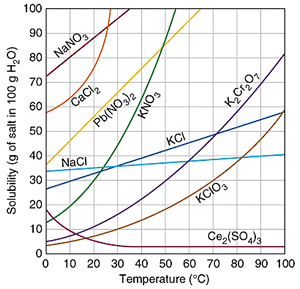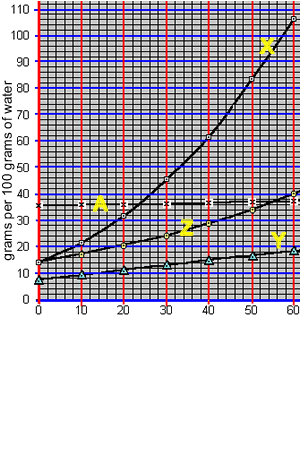Solubility curves A solution can be saturated, unsaturated or supersaturated. Solubility of a substance refers to the maximum amount of substance that can dissolve in a given volume of solvent at a certain temperature. We measure solubility of a substance by measuring the maximum mass of solute that can be dissolved in 100 grams of solvent at a given temperature. The relationship between solubility and temperature can be expressed by a solubility curve. The solubility curves of several compounds are shown on the right.Such curves reveal the maximum amount of solute that can be dissolved in 100 grams of water over a range of temperatures. The solubility of most compounds increases as temperature increases, although exceptions do exist.How do we read the solubility curves? Consider the solubility curves of 4 unknown compounds, shown on the right. Consider compound "X". Reading straight from the solubility curve we can see that at 40 oC the maximum mass of substance "X" that can dissolve in 100 grams of water is 62 grams. 1) What mass of substance "X" will dissolve in 100 grams of water at 30 oC? 36 grams 46 grams 56 grams 32 grams 2) What mass of substance "Y" will dissolve in 100 grams of water at 60 oC? 36 grams 46 grams 22 grams 18 grams 3) At what temperature will 40 grams of substance "X" form a saturated solution when added to 100 grams of water? 26 36 44 20What mass of compound "X" can be dissolved in 50 grams of water at 40 oC? The animation on the right takes us through the working out. At 40 oC, 100 grams of water can only hold 62 grams of compound "X". The ratio of mass of solute to mass of water remains the same at a given temperature. So: at 40 oC => (62 grams / 100 grams) = (x grams / 50 grams) => 31 grams = x1) What is the total mass of compound "X" that can be dissolved in 25 grams of water at 26 oC? 25 10 40 20 Solution 2) What is the total mass of compound "A" that can be dissolved in 50 grams of water at 20 oC? 16 32 40 28 3) What is the total mass of compound "Y" that can be dissolved in 20 grams of water at 60 oC? 2.0 3.6 2.6 18 Continue with precipitation.